# 概述# 5.0 上篇回顾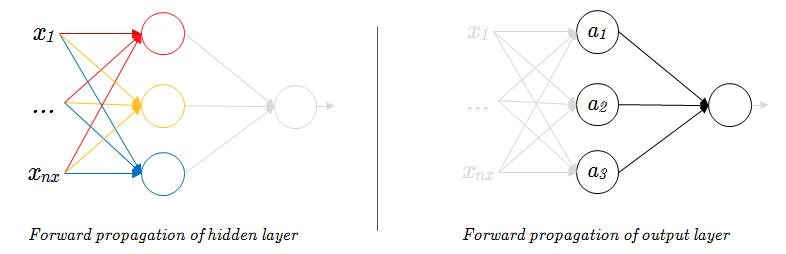sigmoid 函数帮助我们将 Logistic 线性回归值进行归一化处理，得到一个位于区间 $[0,1]$ 的概率输出。但在浅层神经网络以至于在深层神经网络当中，尽管 sigmoid 函数可以继续用于输出层，但往往不会作为隐藏层的激活函数，而是使用其它的一些函数，如 tanh、ReLU、Leaky ReLU 等，这是为什么？下面就来了解一下这些新奇的函数，以及为什么要使用这些函数而不是 sigmoid 函数吧。

# 5.1 激活函数的选择

## tanh

tanh 函数是双曲函数中的一个，更准确的说是双曲正切函数。首先来看一看 tanh 函数，它的函数表达式是这样的：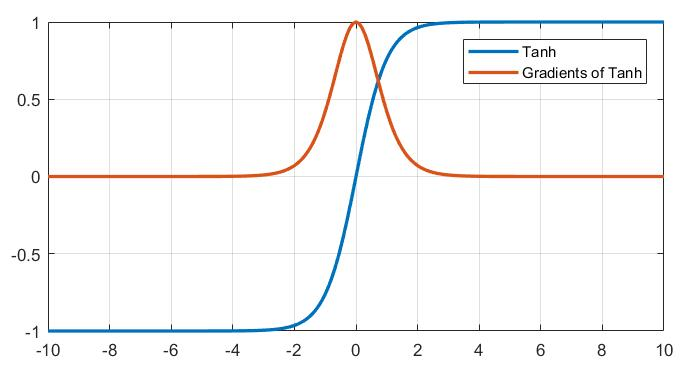## ReLU

ReLU（Rectified Linear Unit），又叫线性整流函数、线性修正单元，是进来神经网络的隐藏层中被普遍选用的激活函数，其表达式如公式(4)所示:

\begin{equation}
ReLU(z)=\left\{
\begin{array}{rcl}
z & & {z>0}\\
0 & & {z<0}
\end{array} \right.
\end{equation}

\begin{equation}
ReLU(z)=max(0,z)
\end{equation}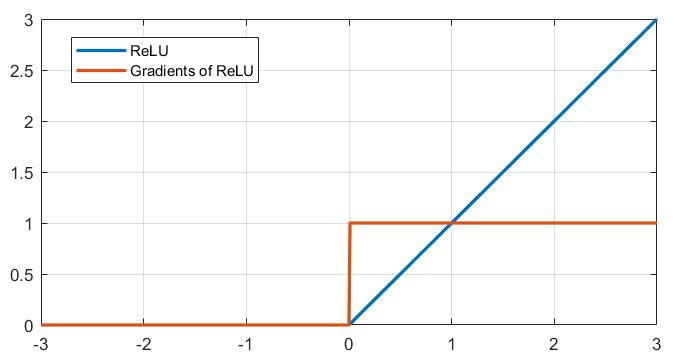\begin{equation}
ReLU(z)=\left\{
\begin{array}{rcl}
z & & {z>0}\\
0 & & {z \leq 0}
\end{array} \right.
\end{equation}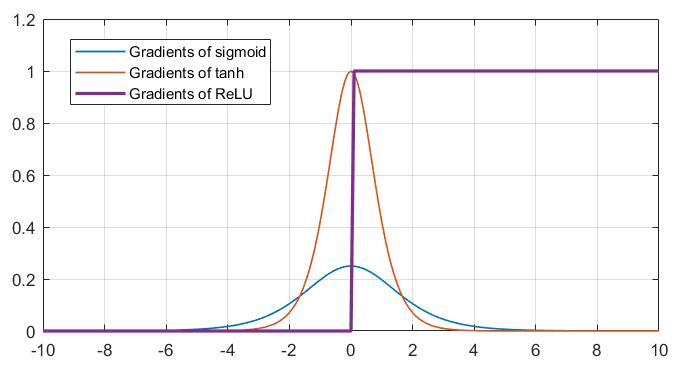——百度百科

## Leaky ReLU

\begin{equation}
LeakyReLU(z)=\left\{
\begin{array}{rcl}
z & & {z>0}\\
\alpha z & & {z<0}
\end{array} \right.
\end{equation}

Leaky ReLU 与 ReLU 的区别仅仅在于当输入量小于零时，引入了一个极小的梯度 $\alpha$（图1-4中取值为0.05），使得神经元不至于被完全抑制，但是这种做法的优劣不好评定，在一些情况下，Leaky ReLU 可能表现更好，另一些情况下可能 ReLU 更胜一筹，谁知道呢。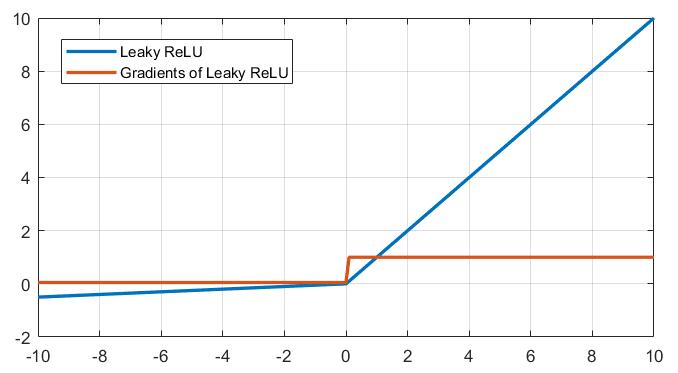﻿
0%